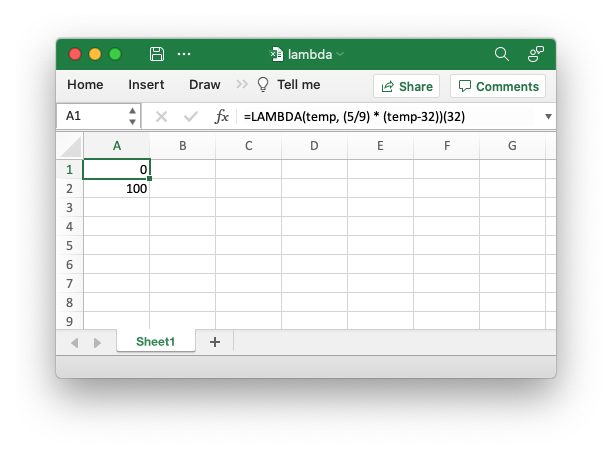libxlsxwriter
lambda.c

Example of using the new Excel `LAMBDA()` function. It demonstrates how to create a lambda function in Excel and also how to assign a name to it so that it can be called as a user defined function. This particular example converts from Fahrenheit to Celsius. Note, this function is only currently available if you are subscribed to the Microsoft Office Beta Channel program./*
* An example of using the new Excel LAMBDA() function with the libxlsxwriter
* library. Note, this function is only currently available if you are
* subscribed to the Microsoft Office Beta Channel program.
*
* Copyright 2014-2021, John McNamara, jmcnamara@cpan.org
*
*/
#include "xlsxwriter.h"
int main() {
lxw_workbook *workbook = workbook_new("lambda.xlsx");
lxw_worksheet *worksheet = workbook_add_worksheet(workbook, NULL);
worksheet_write_string(worksheet, 0, 0,
"Note: Lambda functions currently only work with "
"the Beta Channel versions of Excel 365", NULL);
/* Note that the formula name is prefixed with "_xlfn." and that the
* lambda function parameters are prefixed with "_xlpm.". These prefixes
* won't show up in Excel.
*/
"=_xlfn.LAMBDA(_xlpm.temp, (5/9) * (_xlpm.temp-32))(32)",
NULL);
/* Create the lambda function as a defined name and write it as a dynamic formula. */
"ToCelsius",
"=_xlfn.LAMBDA(_xlpm.temp, (5/9) * (_xlpm.temp-32))");
worksheet_write_dynamic_formula(worksheet, CELL("A3"), "=ToCelsius(212)", NULL);
workbook_close(workbook);
return 0;
}
workbook_close
lxw_error workbook_close(lxw_workbook *workbook)
Close the Workbook object and write the XLSX file.
workbook_new
lxw_workbook * workbook_new(const char *filename)
Create a new workbook object.
worksheet_write_dynamic_formula
lxw_error worksheet_write_dynamic_formula(lxw_worksheet *worksheet, lxw_row_t row, lxw_col_t col, const char *formula, lxw_format *format)
Write an Excel 365 dynamic array formula to a worksheet cell.
lxw_worksheet
Struct to represent an Excel worksheet.
Definition: worksheet.h:2107
workbook_define_name
lxw_error workbook_define_name(lxw_workbook *workbook, const char *name, const char *formula)
Create a defined name in the workbook to use as a variable.
lxw_workbook
Struct to represent an Excel workbook.
Definition: workbook.h:280
worksheet_write_string
lxw_error worksheet_write_string(lxw_worksheet *worksheet, lxw_row_t row, lxw_col_t col, const char *string, lxw_format *format)
Write a string to a worksheet cell.
CELL
#define CELL(cell)
Convert an Excel A1 cell string into a (row, col) pair.
Definition: utility.h:45